### DIFFERENTIATION OF INVERSE TRIGONOMETRIC FUNCTIONS

None of the six basic trigonometry functions is a one-to-one function. However, in the following list, each trigonometry function is listed with an appropriately restricted domain, which makes it one-to-one.
1.for2.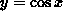for3.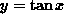for4.for, except5.for, except x = 0
6.forBecause each of the above-listed functions is one-to-one, each has an inverse function. The corresponding inverse functions are
1.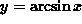for2.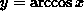for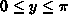3.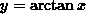for4.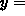arcfor, except5.arcfor, except y = 0
6.arc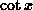forIn the following discussion and solutions the derivative of a function h(x) will be denoted byor h'(x) . The derivatives of the above-mentioned inverse trigonometric functions follow from trigonometry identities, implicit differentiation, and the chain rule. They are as follows.
1.2.3.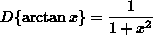4.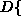arc5.arc6.arc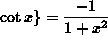In the list of problems which follows, most problems are average and a few are somewhat challenging.

• PROBLEM 1 : Differentiate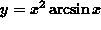.

• PROBLEM 2 : Differentiate.

• PROBLEM 3 : Differentiate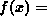arcarc.

• PROBLEM 4 : Letarc. Solve f'(x) = 0 for x .

• PROBLEM 5 : Let. Show that f'(x) = 0 . Conclude that.

• PROBLEM 6 : Evaluate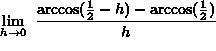.

Some of the following problems require use of the chain rule.

• PROBLEM 7 : Differentiate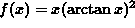.

• PROBLEM 8 : Differentiate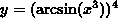.

• PROBLEM 9 : Differentiate.

• PROBLEM 10 : Determine the slope of the line tangent to the graph of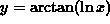at x = e .

• PROBLEM 11 : Differentiatearc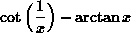. What conclusion can be drawn from your answer about function y ? What conclusion can be drawn about functions arcand?

• PROBLEM 12 : Differentiate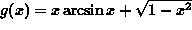.

• PROBLEM 13 : Find an equation of the line tangent to the graph ofat x=2 .

• PROBLEM 14 : Evaluate.

• PROBLEM 15 : A movie screen on the front wall in your classroom is 16 feet high and positioned 9 feet above your eye-level. How far away from the front of the room should you sit in order to have the ``best" view ? (HINT: Find the largest possible anglein the given diagram below.)